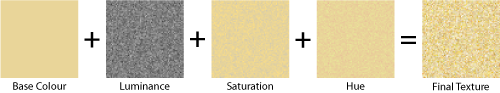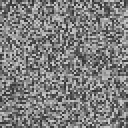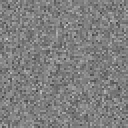## Feb 1, 2011

### Procedural Texture Generation

As I mentioned in the introductory post, Bulldog is going to feature procedural content. This will cover things like terrain, random encounters and even textures.

At present, the only procedural content I've built for Bulldog is a texture generator class which generates textures from pre-defined templates. These templates specify a series of operations performed sequentially to produce the desired output. At present it is fairly naive and produces rudimentary results, but I hope to improve the texture generator's capabilities over time with more advanced synthesis techniques.

I've found that RGB is not a particularly good colour system for texture modelling, as you rarely want to adjust the redness, blueness or greenness of a particular pixel. You're more likely to want to adjust the brightness or other parameters. So I use the HSL colour system, which consists of Hue, Saturation and Luminance. This allows me to adjust the texture in a much more "natural" way.

As an example of how the texture generation process works, consider a sand texture. Sand is a granular solid made up of tiny bits of rock. The grains are vaguely uniform in size, but vary in colour. I've modelled this in my texture generator by taking a sand-like base colour, then applying random noise to each pixel to adjust the hue, saturation and brightness accordingly. The image below shows the different parts that are generated by the texture generator class and combined to form the final texture.Uniform vs Gaussian noise

The random noise function I used in the examples above is called Gaussian Noise. This is noise where the probability distribution follows the "normal" distribution. To generate Gaussian Noise, you need a random number generator that will produce random numbers that are biased in such a way that they follow the normal distribution, rather than a uniform distribution.

The random number generator in .net (found in System.Random), is typical of most random number functions found in modern languages in that it returns random numbers with a uniform probability distribution. This means that each value in the output range chosen has equal probability of being output. If you were to graph the probability of each value in the range appearing, the result would pretty much be a horizontal line.

The "normal" probability distribution on the other hand, has a symmetric, bell-shaped curve. Values around the mean have a higher probability of being output, and values at the very edges of the range have a much lower probability. I won't go into any more depth on the topic, for further reading look at something like
this wikipedia article.

Below are two examples of noise generated using Uniform and Gaussian Noise:Uniform Noise Gaussian Noise

At first glance it appears as though the uniform noise has a wider range, as it has significantly darker and lighter pixels than the gaussian noise. However, if you look closely at the Gaussian noise you can see that there are some pixels that are quite dark, and some that are quite light, however the majority are somewhere in between, ie, clustered around the mean value. Gaussian noise is a closer match for real-world applications, as the normal distribution appears very frequently in the real world.

For those that are interested, to generate the Gaussian random numbers I wrote a random number generator based on the Box-Muller Transform. It uses a standard uniform probability distribution random number generator and then remaps the values so the output has the normal distribution. The actual implementation I chose uses the Marsaglia Polar Method, which eliminates the need for the sin() and cos() functions.

An interesting quirk with this algorithm is that it needs 2 independent uniform random numbers to actually work, but the output is 2 independent normally distributed random numbers, so in my implementation I actually cache the second value and return that without the need for computation on the second call.